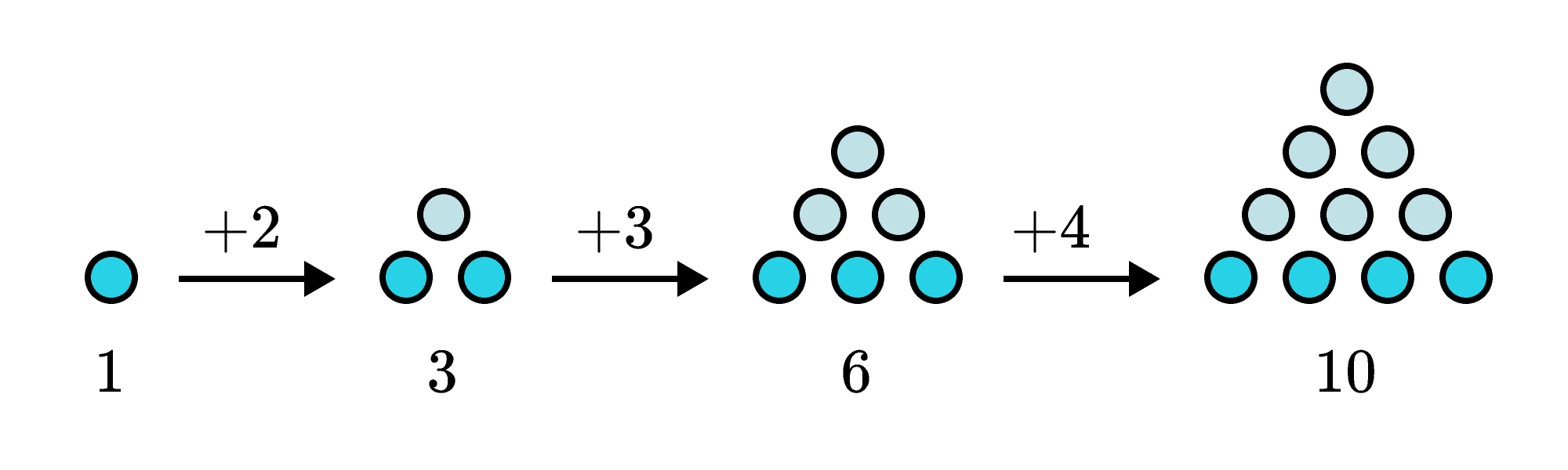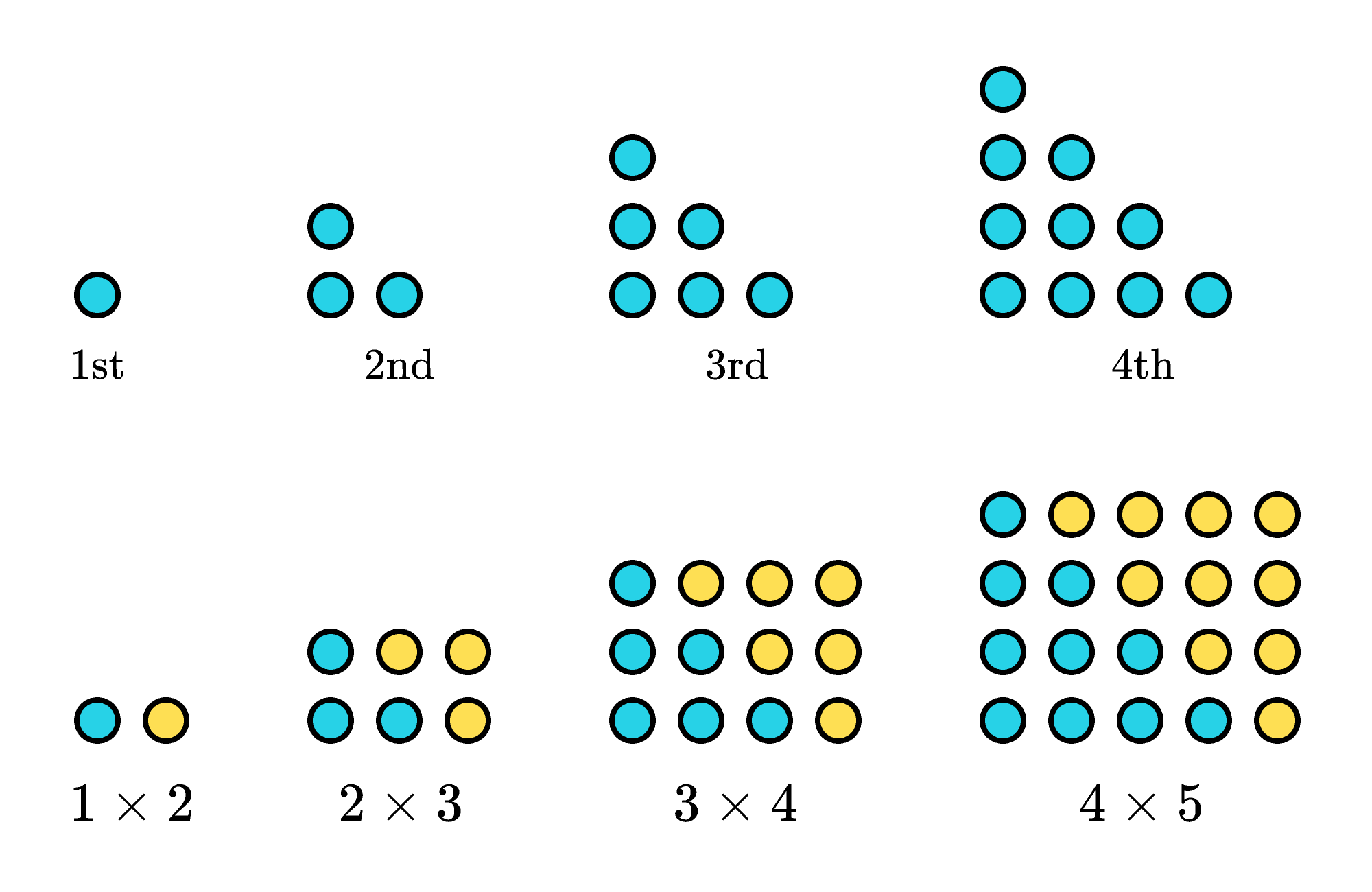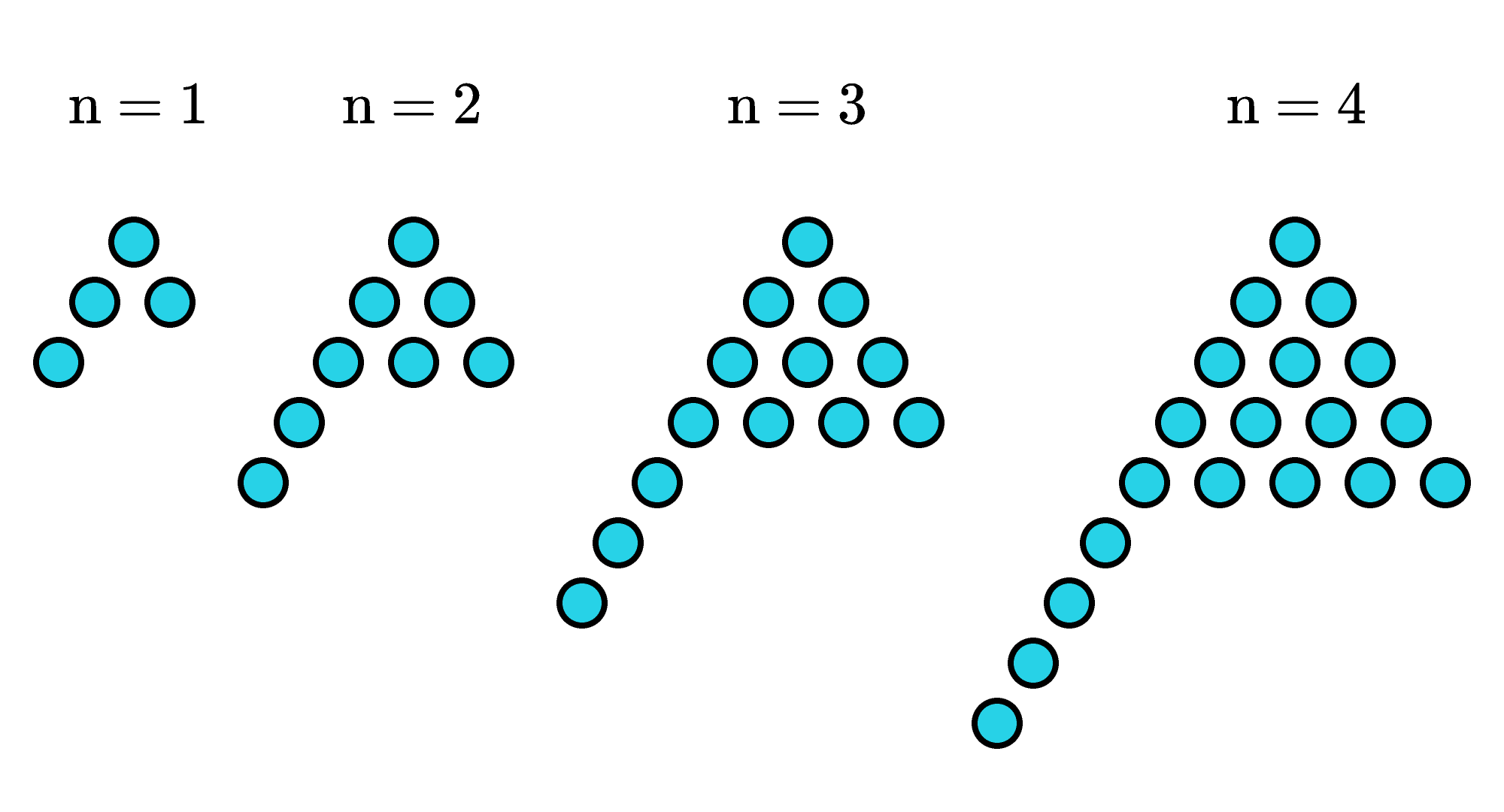## Rearrange the Dots

In the pattern below, we add a new row to the bottom of the triangle with every step. The next term will then be $10+5=15,$ and we can find the one after that by adding six: $15+6=21$.We could extend this pattern as far as we want, making larger and larger triangles, but is it possible to skip straight to the number of dots in the $100^\text{th}$ triangle without calculating all the figures in between?

To find the number of dots in the $100^\text{th}$ triangle, we should look for an explicit formula that describes the number of dots in each figure. However, it’s not immediately obvious how we might describe the number of dots $($apart from the already established pattern of $1 + 2 + 3 + 4 + \cdots).$

If we rearrange and double the triangles, though, it’s possible to make each of them into a rectangular array, which we can then describe algebraically.After we’ve doubled the triangles, each triangle forms a rectangle with these dimensions: one side has the same number of dots as the base of the triangle, while the other side is one unit longer than that.

Since the base of each triangle is $n$ dots, these rectangles have $n(n + 1)$ dots, so the triangles (which are half) have $\frac{n(n + 1)}{2}$ dots.

By rearranging dots, we've made it easier to find a general formula for the number of dots in the $n^\text{th}$ term, which is $\frac{n(n + 1)}{2}$ dots. We can determine that the $100^\text{th}$ term in the sequence has \begin{aligned} \frac{100(100 + 1)}{2} & = \frac{100\times 101}{2} \\ & = \frac{10,100}{2} \\ & = 5,050 \\ \end{aligned} dots.

Can you find the pattern in the dots below?

# Today's Challenge

Which formula describes the dot pattern?×